Matrix ring

(diff) ← Older revision | Latest revision (diff) | Newer revision → (diff)

full matrix ring

The ring of all square matrices of a fixed order over a ring. The ring of-dimensional matrices overis denoted by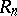or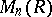. Throughout this articleis an associative ring with identity (cf. Associative rings and algebras).

The ringis isomorphic to the ring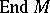of all endomorphisms of the free right-module, possessing a basis withelements. The matrixis the identity in. An associative ringwith identity 1 is isomorphic toif and only if there is ina set of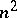elements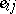,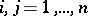, subject to the following conditions:

1)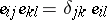,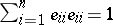;

2) the centralizer of the set of elementsinis isomorphic to.

The centre ofcoincides with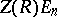, whereis the centre of; forthe ringis non-commutative.

The multiplicative group of the ring(the group of all invertible elements), called the general linear group, is denoted by. A matrix fromis invertible inif and only if its columns form a basis of the free right module of all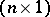-dimensional matrices over. Ifis commutative, then the invertibility of a matrixinis equivalent to the invertibility of its determinant,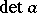, in. The equalityholds.

The ringis simple if and only ifis simple, for the two-sided ideals inare of the form, whereis a two-sided ideal in. An Artinian ring is simple if and only if it is isomorphic to a matrix ring over a skew-field (the Wedderburn–Artin theorem). If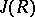denotes the Jacobson radical of the ring, then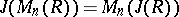. Consequently, every matrix ring over a semi-simple ringis semi-simple. Ifis regular (i.e. if for every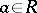there is a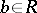such that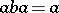), then so is. Ifis a ring with an invariant basis number, i.e. the number of elements in a basis of each free-module does not depend of the choice of the basis, thenalso has this property. The ringsandare equivalent in the sense of Morita (see Morita equivalence): The category of-modules is equivalent to the category of-modules. However, the fact that projective-modules are free does not necessarily entail that projective-modules are free too. For instance, ifis a field and, then there exist finitely-generated projective-modules which are not free.